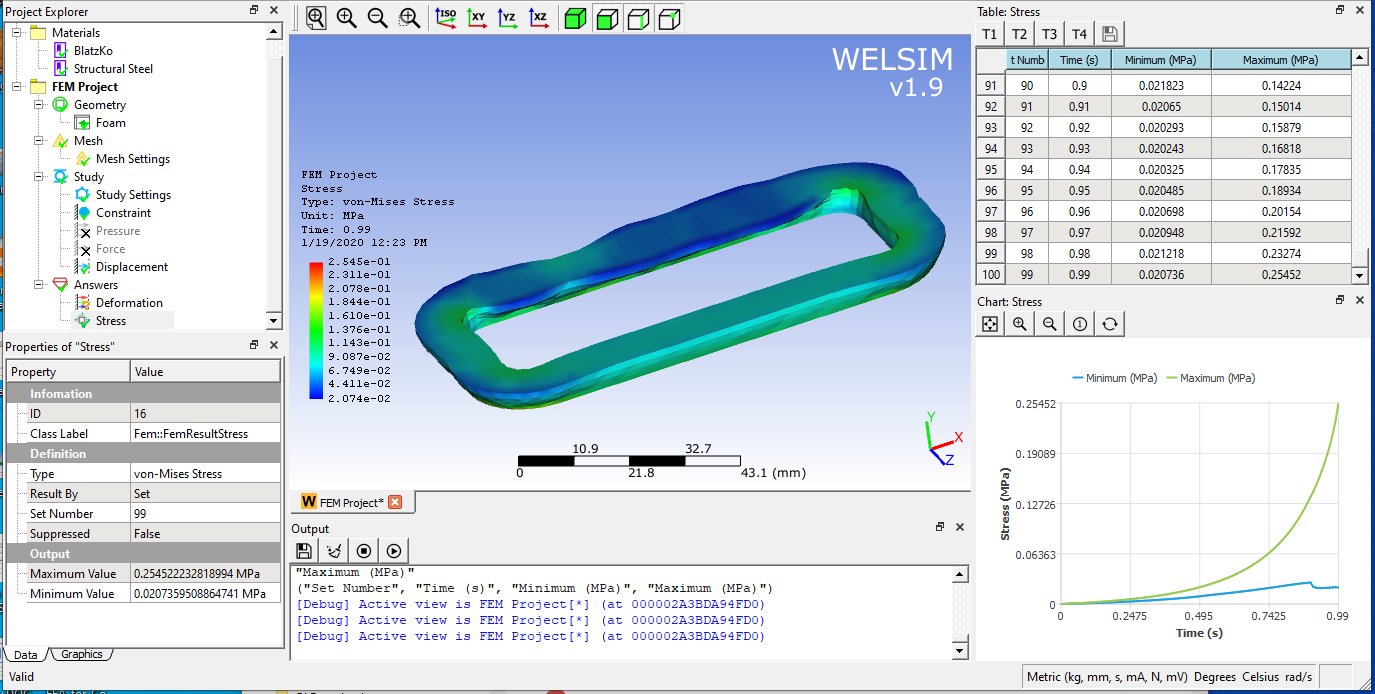# Blatz-Ko hyperelastic model for nonlinear finite element analysis

June 13, 2021

We have introduced the hyperelastic models commonly used in finite element analysis such as Arruda-Boyce, neo-Hookean, Mooney-Rivlin, Yeoh, and Gent. In this article, we will be discussing a very special hyper-elastic model: Blatz-Ko. The most hyperelastic models are mainly designed for incompressible materials, while Blatz-Ko is very suitable for modeling the compressible hyperelastic materials, such as the foamed rubber. Because of the simplicity of math form, Blatz-Ko has been one of the most widely used constitutive models for compressible isotropic nonlinearly elastic solids. Blatz-Ko model is named after Dr. Blatz and Dr. Ko, for their contribution to this hyperelastic model.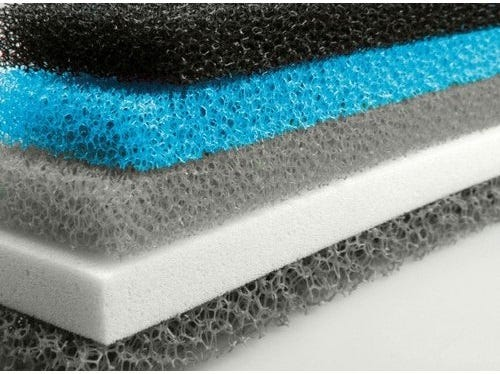In 1962, Dr. Paul J. Blatz and his Ph.D. student William Ko published a lengthy paper entitled “Application of Finite Elastic Theory to the Deformation of Rubbery Materials” in the journal “Transactions of Society of rheology”, describing Blatz- Ko model and its experimental basis. WELSIM already supports the Blatz-Ko hyperelastic model. It provides a simple, accurate and effective mechanical model for compressible hyperelastic materials such as foamed polyurethane rubber. It was also said that Blatz-Ko can be applied for the solid fuel of the rocket.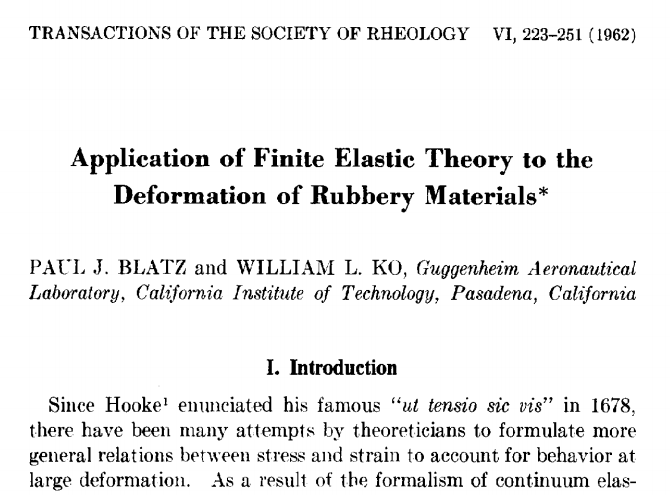Porous rubber or elastic foam materials are generally referred to as foam materials. Common examples of elastic foam materials are porous polymers such as sponges, packaging materials, etc. Foam rubber can go through very large elastic strain. The strain can reach 500% and 90% when the material is tensiled or compressed, respectively. Compared with the almost incompressibility of solid rubber, the porosity of the foam material allows very large volume-reduced deformations and therefore has good energy absorption. At the small strain condition (less than 5%), the material shows linear elasticity with Poisson’s ratio of 0.3 or so. At large strain deformation, the Poisson’s ratio of the material goes to 0.0 when it is compressed and becomes larger than zero when it is stretched. These special mechanical properties are very suitable to be described by the Blatz-Ko model.

Blatz-Ko’s strain-energy potential has a general form covering lower- and higher-order versions. Its lower-order form is most commonly used in finite element methods. It is given as follows: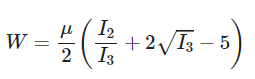Where u is the initial shear modulus, I2 and I3 are the second and third invariants of the strain tensor, respectively. Since volumetric and deviatoric parts are strongly coupled, the user does not need to input incompressibility parameters. The bulk modulus can be determined with shear modulus. It is worth noting that I2 and I3 here are the original invariants, not reduced invariants that often used for other hyperelastic models. From the expression of the strain energy function, we know:

1. There is only one input parameter: the initial shear modulus u.
2. The initial bulk modulus is K = 5u / 3. Volumetric and deviatoric terms are strongly coupled. This is fundamentally different from other hyper-elastic models.

### Behaviors and limitations of the Blatz-Ko:

1. Simple. Only one input parameter. Therefore, the amount of the required experiment is small.
2. It can show the mechanical behavior of materials with small Poisson’s ratio.
3. Like all other low-order or two/three-parameter models, Blatz-Ko is unable to predict the whole range of strain.

### Finite element analysis with Blatz-Ko material model

Define materials Select Blatz-Ko hyperelastic material, and set the parameter u = 0.01 MPa.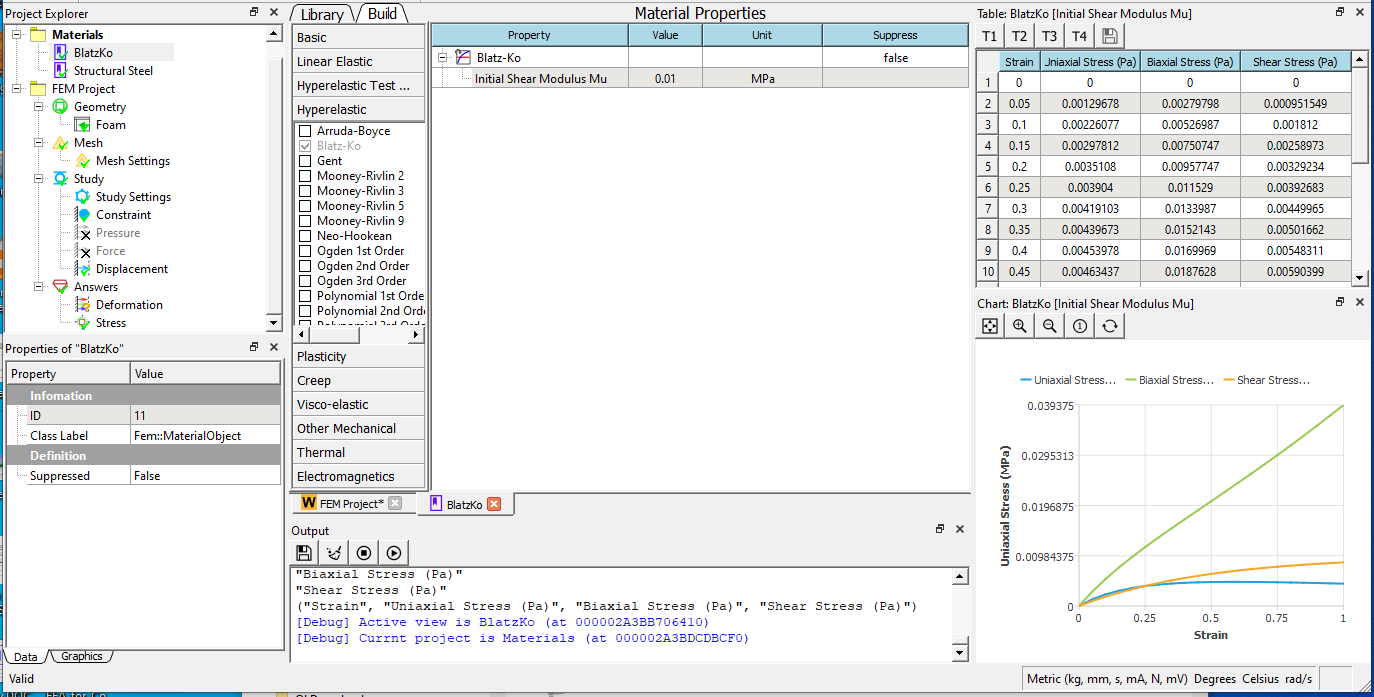Import the foam geometry, mesh it, and apply a downward displacement of -7mm.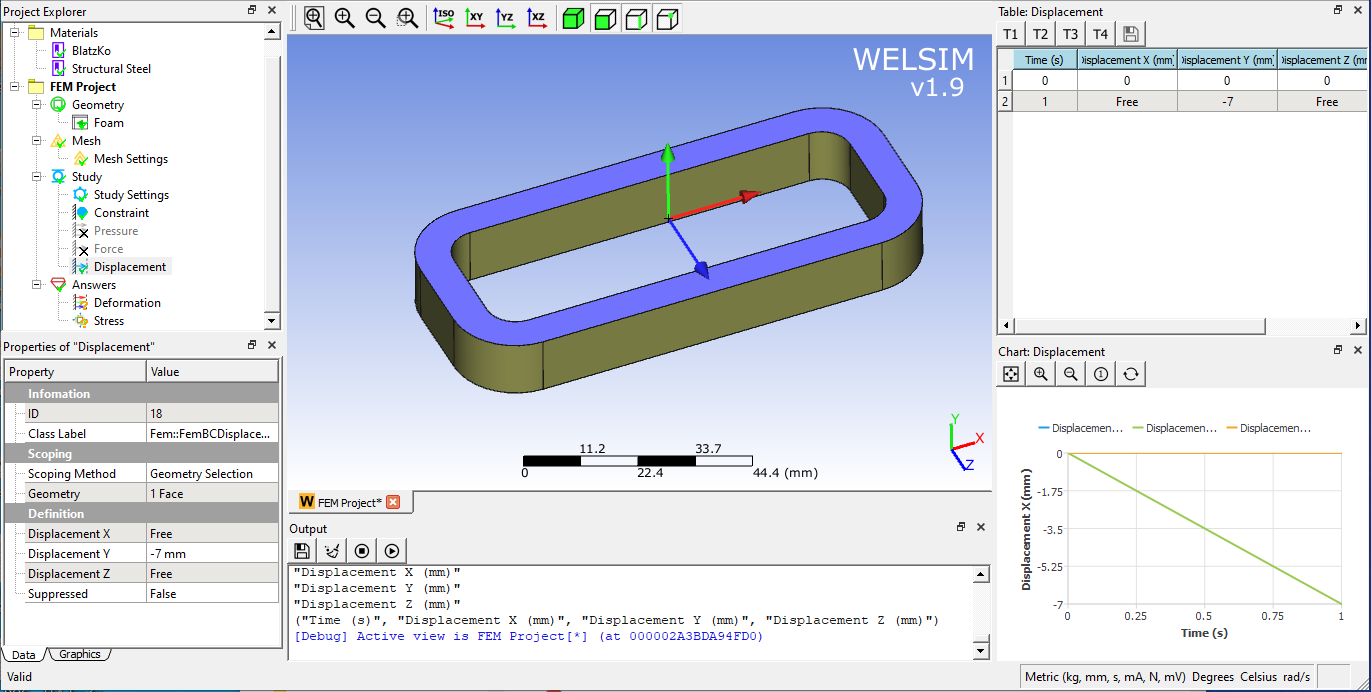Solve and add stress result object to show the von-Mises stress contour. As shown in the figure, the maximum stress value is non-linear with regards to time.